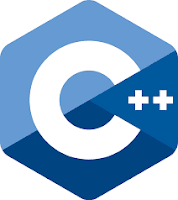Constructor In C++ | Program Examples - LBM4

## Friday, 29 November 2019A constructor is basically a function used for initialization of the class data members, allocation of memory, and so on. It is convenient if an object can initialize itself when it is first created, without the need to make a separate call to a member function. It has the same name as the class in which they are members. No return type is used for constructors. Since the constructor is called automatically by the system there is no reason for it to return anything. Compiler knows that they are constructors by looking at their name and return type. Let us take an example that each time an object of the date class is created, it is to be initialized with the date 2/02/2011.

Examples:

class date{
private:
int dd,yy;
char mm;
public:
date() // constructor
{ dd=2,mm='0',mm='2';
mm='\0', yy=2011;
}
showdate() //display
{
cout<<"\n"<<dd<<"/"<<mm<<"/"<<yy;
}
};
int main()
{
date d1; //define and initialize
clrscr();
d1.showdate();
return 0;
}

Write a program that has a class to represent time. The class should have constructors to initialize data members hour, minute and second to 0 and to initialize them to values passed as arguments. The class should have member function to add time objects and return the result as time object. There should be another function to display the result in 24 hour format

``` #include<iostream>
using namespace std;
class date
{
int hr,meen,sec;
public:
date()
{
hr=0;
meen=0;
sec=0;
}
date(int a, int b, int c)
{
hr=a;meen=b; sec=c;
}
{
date d3;
d3.sec=d1.sec+d2.sec;
d3.meen=d1.meen+d2.meen+d3.sec/60;
d3.sec=d3.sec%60;
d3.hr=d1.hr+d2.hr+d3.meen/60;
d3.meen=d3.meen%60;

return d3;

}
void display()
{
cout<<"hour"<<hr<<endl;
cout<<"min"<<meen<<endl;
cout<<"sec"<<sec<<endl;
}
};
main()
{
date d1(10,40,80),d2(12,34,56),d3;
d3.display();
return 0;
}
```
Write a program that has a class with a dynamically allocated character array as its data member. One object should contain "Engineers are" and another should contain " Creatures of logic". Member function join() should concatenate two strings by passing two objects as arguments. Display the concatenated string through a member function. Use constructors to allocate and initialize the data member. Also, write a destructor to free the allocated memory for the character array. Make your own function for concatenation of two strings.
```
#include<iostream>

#include<cstring>
using namespace std;
class dyna
{
char*arr;
public:
dyna(char*input)
{
arr=new char[strlen(input)];
strcpy(arr,input);
}
void join(dyna&a,dyna&b )
{
int l1=strlen (a.arr);
int l2=strlen (b.arr);
delete []arr;
arr=new char [l1+l2+1];
int i=0;

while(l1<l2)
{
arr [i]=a.arr[i];
i++;
}
while(i<l1+l2)
{
arr[i]=b.arr[i-l1]
++i;
}
arr[l1+l2]='\0';
}
void show()
{
cout<<arr<endl;
}
~dyna()
{
delete[]arr;
}

};
main()
{
char c1[]="Engineers are";
char c2[]="creatures of logic";
char c3[]=" ";
dyna lt(c1);
dyna yt(c2);
dyna t1(c3);
t1.join(lt,yt);
t1.show();
return 0;

}```